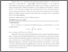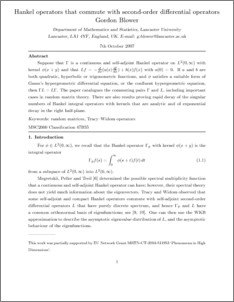# Hankel operators that commute with second order differential operators.

Blower, Gordon (2008) Hankel operators that commute with second order differential operators. Journal of Mathematical Analysis and Applications, 342 (1). pp. 601-614. ISSN 0022-247XPreview
PDF (integrablepaper3.pdf)
integrablepaper3.pdf

Suppose that $\Gamma$ is a continuous and self-adjoint Hankel operator on $L^2(0, \infty )$ with kernel $\phi (x+y)$ and that $Lf=-(d/dx)(a(x)df/dx)+b(x)f(x) with$a(0)=0$. If$a$and$b$are both quadratic, hyperbolic or trigonometric functions, and$\phi$satisfies a suitable form of Gauss's hypergeometric differential equation, or the confluent hypergeometric equation, then$\Gamma L=L\Gamma\$. There are also results proving rapid decay of the singular numbers of Hankel integral operators with kernels that are analytic and of exponential decay in the right half-plane.View Item